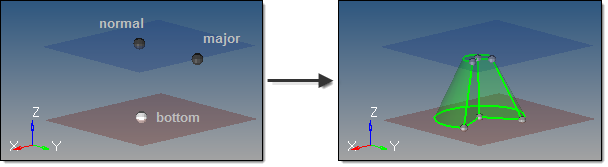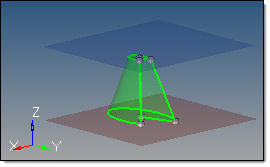﻿ cone partial subpanel (Solids panel)
Manufacturing Solutions

# cone partial subpanel (Solids panel)# cone partial subpanel (Solids panel)This subpanel creates three-dimensional, partial-cone solid primitives.Five inputs are required to create a cone using this method:

 • The bottom center node defines the center of the bottom cone face.
 • The vector between the bottom center node and the normal vector node defines the cone axis, thereby indicating the cone orientation.  This does not indicate the actual cone height.
 • The vector between the bottom center node and the major vector node determines the zero-degree point of an arc defining the curved surface of the partial cone.  This arc extends in a direction based on the normal vector using the right-hand rule, with its start angle and end angle specified relative to this vector.
 • The top radius defines the radius of the top cone face.  If set to 0, a cone tip is created; if greater than zero, the cone has a flat top.
 • The base radius defines the radius of the bottom cone face.  Must be greater than 0.
 • The cone height defines the distance between the cone's base and its top.
 • The start angle defines the starting arc angle, measured from the major vector node in a direction based on the normal vector using the right-hand rule.
 • The end angle defines the ending arc angle, measured from the major vector node in a direction based on the normal vector using the right-hand rule.  The difference between this and the start angle determines the arc of the partial cone, and therefore the arc of the cutout in the partial cone.  For example, if your start angle is 15 degrees, and your end angle is 285 degrees, the resulting cone has a base with a 270 degree arc and a 90 degree cut.
 • The axis ratio represents a percentage of the major vector.  This value must be greater than zero and less than or equal to 1.  Decimal values create oval-shaped cones instead of circular ones.